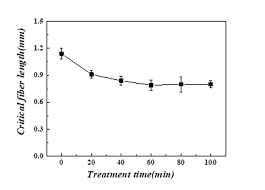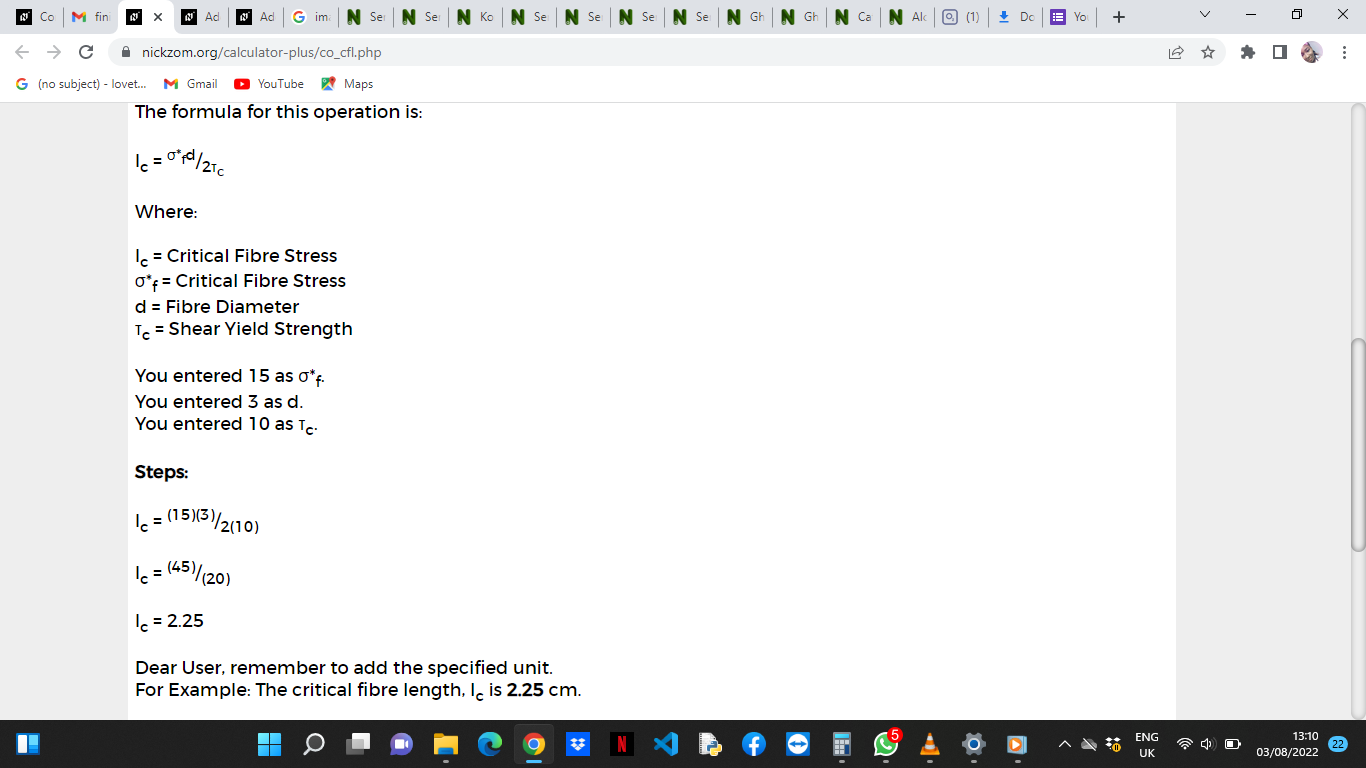# How to Calculate and Solve for Critical Fibre Length | Composites

The critical fibre length is illustrated by the image below.To compute for critical fibre length, three essential parameters are needed and these parameters are Critical Fibre Stress (σ*f), Fibre Diameter (d) and Shear Yield Strength (τc).

The formula for calculating critical fibre length:

lc = σ*fd/2τc

Where:

lc = Critical Fibre Length
σ*f = Critical Fibre Stress
d = Fibre Diameter
τc = Shear Yield Strength

Let’s solve an example;
Find the critical fibre length when the critical fibre stress is 15, the fibre diameter is 3 and the shear yield strength is 10.

This implies that;

σ*f = Critical Fibre Stress = 15
d = Fibre Diameter = 3
τc = Shear Yield Strength = 10

lc = σ*fd/2τc
lc = (15)(3)/2(10)
lc = (45)/(20)
lc = 2.25

Therefore, the critical fibre length is 2.25 cm.

Calculating the Critical Fibre Stress when the Critical Fibre Length, the Fibre Diameter and the Shear Yield Strength is Given.

σ*f = lc x 2τc / d

Where:

σ*f = Critical Fibre Stress
lc = Critical Fibre Length
d = Fibre Diameter
τc = Shear Yield Strength

Let’s solve an example;
Given the critical fibre length as 24, the fibre diameter as 12 and the shear yield strength as 4. Find the critical fibre stress?

This implies that;

lc = Critical Fibre Length = 24
d = Fibre Diameter = 12
τc = Shear Yield Strength = 4

σ*f = lc x 2τc / d
σ*f = 24 x 2(4) / 12
σ*f = 24 x 8 / 12
σ*f = 192 / 12
σ*f = 16

Therefore, the critical fibre stress is 16.

Calculating the Fibre Diameter when the Critical Fibre Length, the Critical Fibre Stress and the Shear Yield Strength is Given.

d = lc x 2τc / σ*f

Where:

d = Fibre Diameter
lc = Critical Fibre Length
σ*f = Critical Fibre Stress
τc = Shear Yield Strength

Let’s solve an example;
Find the fibre diameter when the critical fibre length is 20, the critical fibre stress is 32 and the shear yield strength is 12.

This implies that;

lc = Critical Fibre Length = 20
σ*f = Critical Fibre Stress = 32
τc = Shear Yield Strength = 12

d = lc x 2τc / σ*f
d = 20 x 2(12) / 32
d = 20 x 24 / 32
d = 480 / 32
d = 15

Therefore, the fibre diameter is 15 cm.

Calculating the Shear Yield Strength when the Critical Fibre Length, the Critical Fibre Stress and the Fibre Diameter is Given.

τc = σ*fd / lc x 2

Where:

τc = Shear Yield Strength
lc = Critical Fibre Length
σ*f = Critical Fibre Stress
d = Fibre Diameter

Let’s solve an example;
Given that the critical fibre length is 14, the critical fibre stress is 8 and the fibre diameter is 6. Find the Shear Yield Strength?

This implies that;

lc = Critical Fibre Length = 14
σ*f = Critical Fibre Stress = 8
d = Fibre Diameter = 6

τc = σ*fd / lc x 2
τc = (8)(6) / 14 x 2
τc = 48 / 28
τc = 1.714

Therefore, the shear yield strength is 1.714.

Nickzom Calculator – The Calculator Encyclopedia is capable of calculating the critical fibre length.

To get the answer and workings of the critica fibre length using the Nickzom Calculator – The Calculator Encyclopedia. First, you need to obtain the app.

You can get this app via any of these means:

You can also try the demo version via https://www.nickzom.org/calculator

Apple (Paid) – https://itunes.apple.com/us/app/nickzom-calculator/id1331162702?mt=8
Once, you have obtained the calculator encyclopedia app, proceed to the Calculator Map, then click on Materials and Metallurgical under Engineering.Now, Click on Composites under Materials and MetallurgicalNow, Click on Critical Fibre Length under CompositesThe screenshot below displays the page or activity to enter your values, to get the answer for the critical fibre length according to the respective parameter which is the Critical Fibre Stress (σ*f), Fibre Diameter (d) and Shear Yield Strength (τc).Now, enter the values appropriately and accordingly for the parameters as required by the Critical Fibre Stress (σ*f) is 15, Fibre Diameter (d) is 3 and Shear Yield Strength (τc) is 10.Finally, Click on CalculateAs you can see from the screenshot above, Nickzom Calculator– The Calculator Encyclopedia solves for the critical fibre length and presents the formula, workings and steps too.